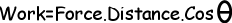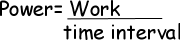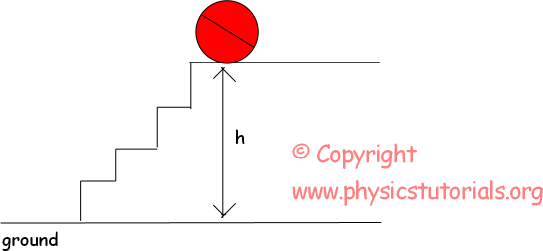## Work Power Energy Cheatsheet

Work Power Energy Cheat Sheet

Work

A force is applied an object and object moves in the direction of applied force then we said work has done. Be careful, direction of applied net force and direction of motion must be same. From our formula we found unit of work kg.m²/s² however, instead of this long unit we use joule. In other words;

1 joule=1N.1mPower

Power is the rate of work done in a unit of time.The unit of the power from the equation given above, joule/s, however, we generally use the unit of power as watt.

1joule/s=1watt

ENERGY

The capability of doing work is called energy. If something has energy then it can do work. It has the same unit with work joule. Mechanical energy is the sum of potential energy and kinetic energy of the system. Let’ see them one by one.

Potential Energy

Objects have energy because of their positions relative to other objects. We call this energy as potential energy. The energy of the objects due to their positions with respect to the ground is called gravitational potential energy.PE=mg.h

By compressing the spring or stretching it you load a potential energy to it. Potential energy of the spring is found with the following formula;

Ep=1/2.k.x²

Where k is the spring constant and x is the amount of compression.

Kinetic Energy

Objects have energy because of their motion; this energy is called kinetic energy. Kinetic energy of the objects having mass m and velocity v can be calculated with the formula given below;

Ek=1/2mv²

The unit of the Ek is again from the formula kg.m²/s² or in general use joule.

Conservation of Energy Theorem

Energy of the systems is always constant, they can change their forms but amount of total energy does not change. For example, in the case of dropping a ball from a height, its potential energy decreases as it falls but its kinetic energy increases with the same time. Potential energy is converted to the kinetic energy and total energy of the system will be conserved.

Einitial = Efinal

Work Power Energy Exams

Author: